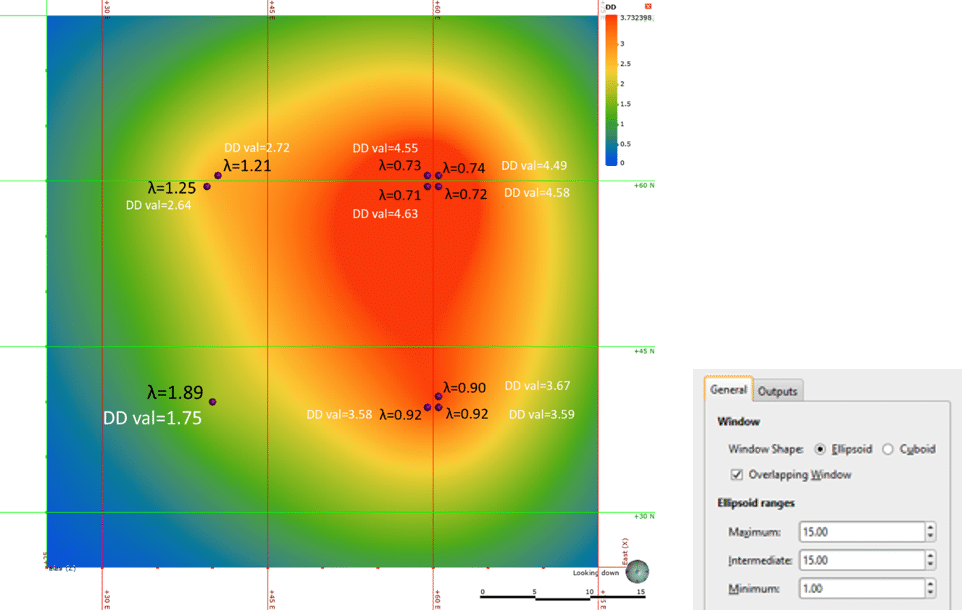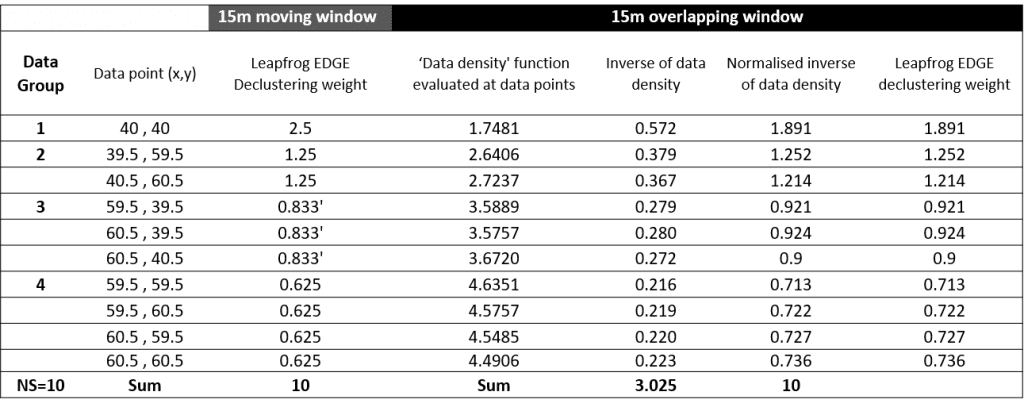Ask many resource practitioners about declustering, and the chances are they will shake their head and mutter something about it being a ‘dark art’.

The purpose of this blog is to describe the algorithms that underlie the Decluster Tool in Leapfrog Edgeto help you to use it effectively.

Leapfrog provides two related, but quite different methods of calculating declustering weights – moving window and overlapping window. The default method is moving window. Overlapping window is invoked by ticking this box.

Conventional Moving Window approach

The first approach Leapfrog provides is a conventional ‘moving window’ declustering approach. This is implemented in a two step process.

Step 1. Calculate the relative (spatial) ‘sampling density’. This is done by centering a search volume onto a desired evaluation point and making a count of the data points that fall inside of the search volume.

Step 2. Calculate ‘declustering weights’. This is done by inverting the count made at each data point, then normalising these to sum to n (the number of data points in the set). When normalised over a set of sample data, the average declustering weight is 1. Weights could equally be normalised to sum to 1, but with a large data set this can result in very small delustering weight values which are hard to visualise. Note that calculation of the ‘sampling density’ is not restricted to data locations, it can be evaluated at any point.

Both steps are illustrated in Figure 1, using a simple 2-dimensional example. In Figure 1 an artificially clustered data set is shown, with 4 groups of data containing 1,2,3 & 4 closely spaced data points respectively. A circular search of 15m has been used to generate a ‘declustering object’. This has been evaluated on to data locations to calculate declustering weights, and onto both data locations, and onto an underlying 50x50m grid with cells of 0.2m. The coloured map shows the result of the evaluation onto the grid.

It can be clearly seen that at each of the data locations, the calculated declustering weight is the normalised inverse of the count of samples (NS) at that location. When evaluated onto the fine underlying grid, the ‘data density’ is equal to the number of samples (ns) falling into the search at any given point.

The radius of the search (15m) is clearly seen around each ‘group’ of data points, as well as the regions where the distance to samples overlaps and the number of samples found in the search differs. The highest count is found in the centre of the plot, where the window overlap all 4 groups of samples, and finds all 10 samples. Note, the boundaries between regions are discreet and sharp, reflecting when samples move into/out of the search distance. There are stripes around the 15m radiuses caused by the small distances between points in the groups of data.Figure 1. Simple 2 dimensional example of a moving window declustering, using an ellipsoidal (circular) search of 15m.  Data points are represented by Purple dots. Number of samples (NS) and declustering weights at each sample location are shown in black text (summarised for each group of points). The coloured map shows the underlying data density, with the DD value and number of samples (ns) shown in white text.

Overlapping Window approach

The second method of declustering that Leapfrog provides uses a different method for calculation of the underlying data density function. In the previous figure, it was seen that the conventional moving window approach results in discontinuities in the underlying data density. While not as apparent as when calculated on drillholes, the stepped nature of the data density still affects the calculation of declustering weights.

This motivated us to develop an alternative approach, to calculate the underlying data density function that eliminates the discontinuities. This is achieved by averaging the point count over all possible windows that contain the evaluation location. In practice an analytical decomposition method that incorporates a spatial weighing function is used, such that the contribution of data points to the ‘data density’ decays as a function of distance. For the ellipsoidal search, the function is equivalent to the well-known spherical covariance function, with a range of twice the specified ellipsoidal ranges.

The difference in how the spatial weighting is applied in the conventional moving window approach and the smoothed overlapping window is illustrated below.

Once again, the most useful way to illustrate the underlying ‘spatial data density’ and the use of that to calculate the declustering weights, is with a simple 2-dimensional example. Figure 3 shows the same artificially grouped data as in Figure 1. The only difference in the creation of the declustering object is that the option for ‘overlapping window’ is ticked.

It is obvious that the resultant data density function is quite different in nature, being smoothed and continuous. Note the difference (compared to Figure 1) of the data density values when evaluated onto data points (think, weighted average sample count). This is because the size of the zone of influence (30m) is twice the size of that used in the moving window, and now spans the distance between sample groups (20m), so that adjacent sample groups are contributing to the sample count.

The direct relationship between the underlying ‘data density’ function and the resultant declustering weights is shown in Table 1.Figure 3. Simple 2-dimensional example of ‘overlapping’ moving window declustering, using an ellipsoidal search. The specified range is 15m (same as Figure 1), but the effective radius over which samples have influence is twice that. The smoothed map shows the resulting data density function evaluated onto a fine block model. The white text shows the values of that function evaluated onto data points, while the black text shows the resultant declustering weights.Table 1. Illustration of calculation of declustering weights from data density for overlapping window, and comparison to moving window.

This blog should demystify the two different methods of calculating declustering weights in Leapfrog EDGE.

Keep an eye out for Part 2 of this blog, where we give guidance on how to make effective use of our declustering tool.